# 【Computer Vision】图像数据预处理详解.

.

.

.# 一、单通道、多通道图像读取

1. 单通道图：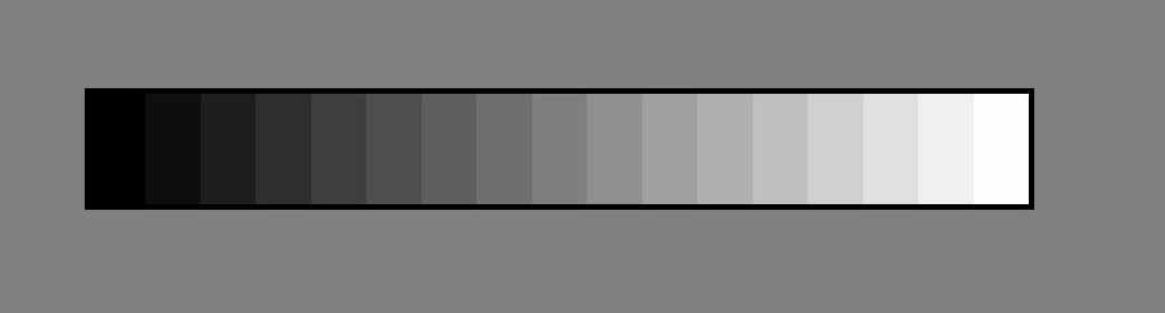1. 三通道图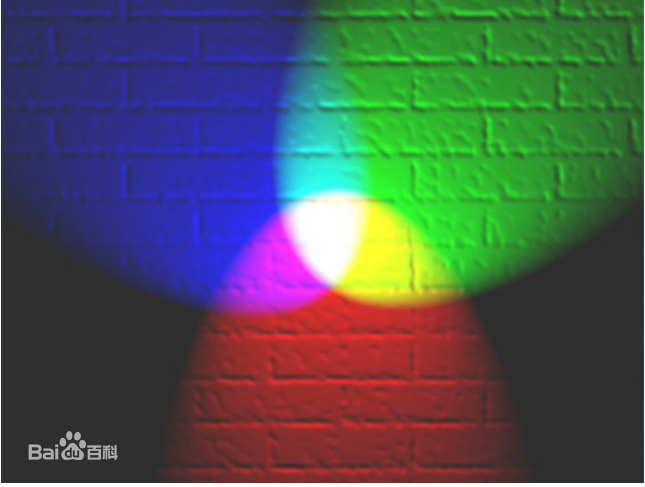## (一)、单通道图像

1. 引入依赖包
``````%matplotlib inline
import numpy as np
import cv2
import matplotlib.pyplot as plt
from PIL import Image
``````
1. 读取单通道图像
``````img = Image.open('lena-gray.jpg')
``````
1. 展示图片
``````display(img)
print(img)
print(img.size)
``````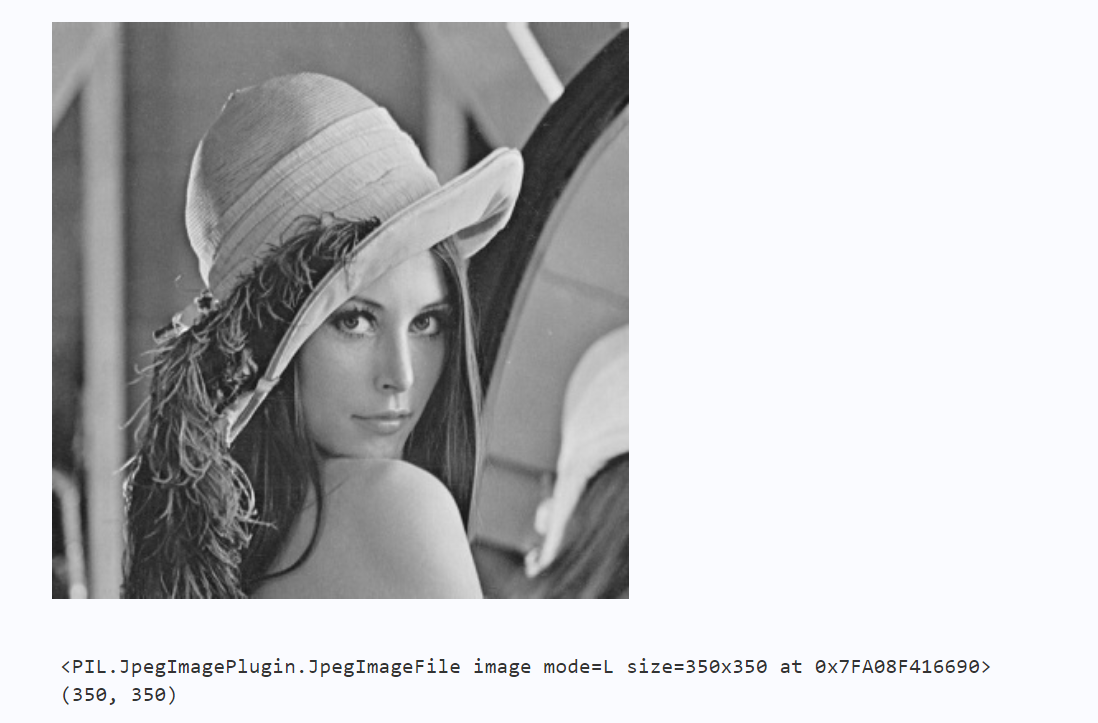## (二)、图像大小与像素值输出

1. 将图片转为矩阵表示
``````# 将图片转为矩阵表示
img_np = np.array(img)
print("图像尺寸：", img_np.shape)
print("图像矩阵：\n", img_np)

``````
1. 将矩阵保存成文本，数字格式为整数
``````# 将矩阵保存成文本，数字格式为整数
# np.savetxt('lena_gray.txt', np.array(img), fmt='%4d')
np.savetxt('lena_gray.txt', img, fmt='%4d')
``````
1. 三通道图像

1. 读取彩色图像
``````img = Image.open('lena.jpg')
# 将图片转为矩阵表示
img_np = np.array(img)
print("图像尺寸：", img_np.shape)
print("图像矩阵：\n", img_np)
``````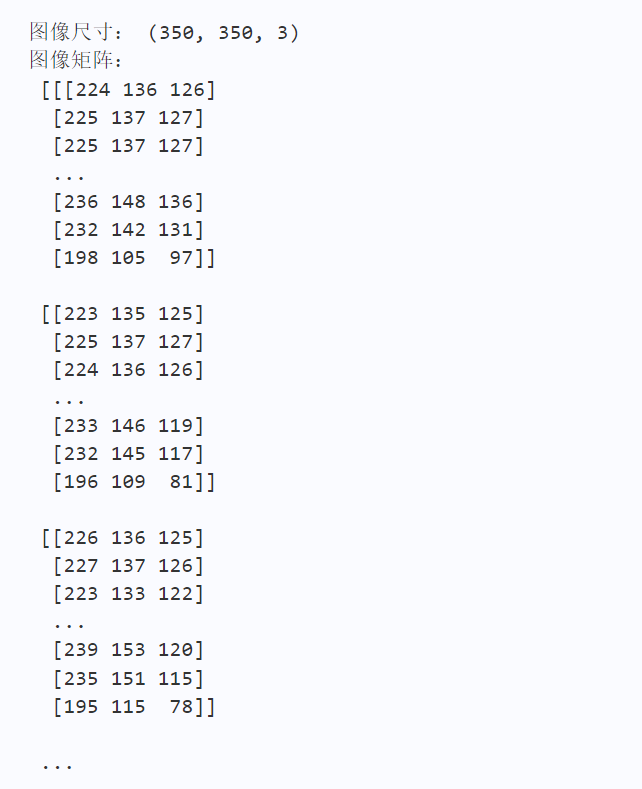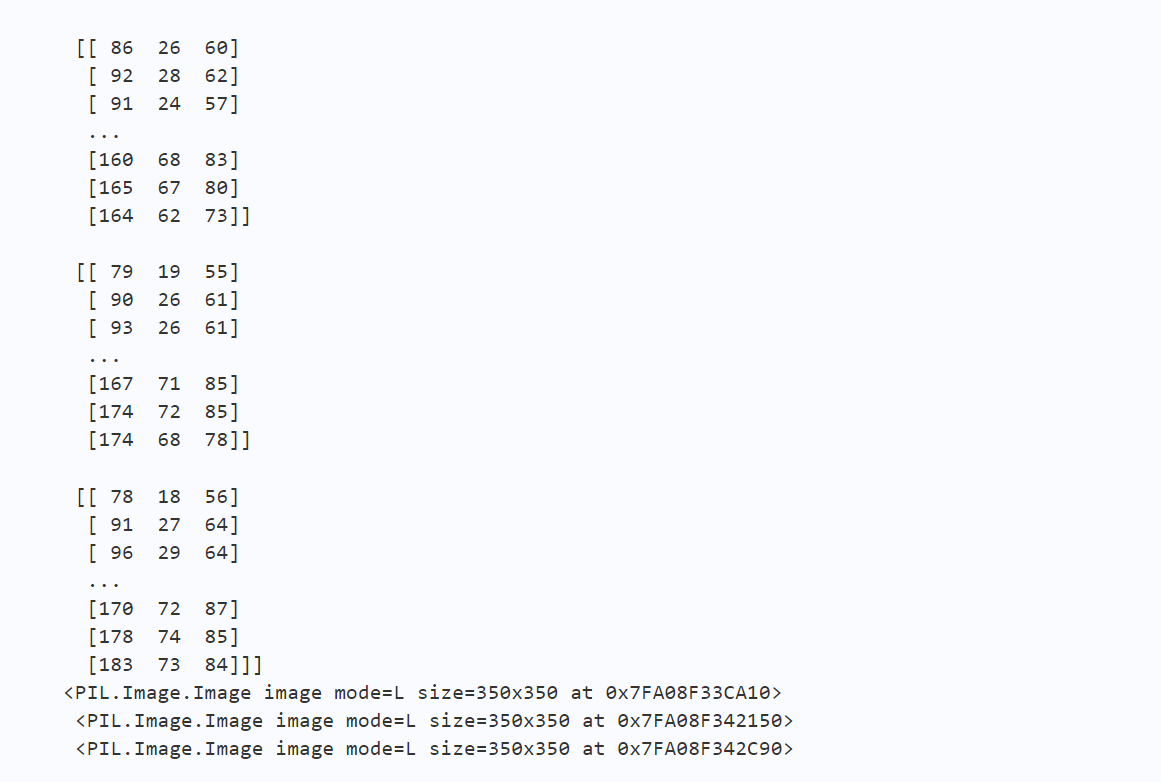## (三)、三通道图像读取

1. 读取彩色图像
``````# 使用PIL分离颜色通道
r,g,b = img.split()
``````

2.获取r通道转的灰度图

``````# 获取r通道转的灰度图
print(r,'\n',g,'\n',b)
print(type(r))
``````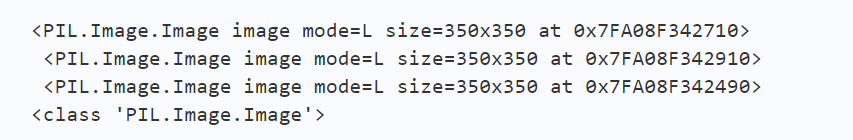1. 展示各通道图像
``````# 获取第一个通道转的灰度图
r = img.getchannel(0)
# 获取第二个通道转的灰度图
display(img.getchannel(1))
# 获取b通道转的灰度图
b
# 获取第二个通道转的灰度图
display(img.getchannel(2))
# 将矩阵保存成文本，数字格式为整数
np.savetxt('lena-r.txt', r, fmt='%4d')
np.savetxt('lena-g.txt', g, fmt='%4d')
np.savetxt('lena-b.txt', b, fmt='%4d')
``````## (四)、使用cv2.split()分离颜色通道

``````# 引入依赖包
%matplotlib inline
import cv2
import matplotlib.pyplot as plt

# 通道分割
b, g, r = cv2.split(img)

# 通道合并
RGB_Image=cv2.merge([b,g,r])
RGB_Image = cv2.cvtColor(RGB_Image, cv2.COLOR_BGR2RGB)
plt.figure(figsize=(12,12))
#显示各通道信息
plt.subplot(141)
plt.imshow(RGB_Image,'gray')
plt.title('RGB_Image')
plt.subplot(142)
plt.imshow(r,'gray')
plt.title('R_Channel')
plt.subplot(143)
plt.imshow(g,'gray')
plt.title('G_Channel')
plt.subplot(144)
plt.imshow(b,'gray')
plt.title('B_Channel')
``````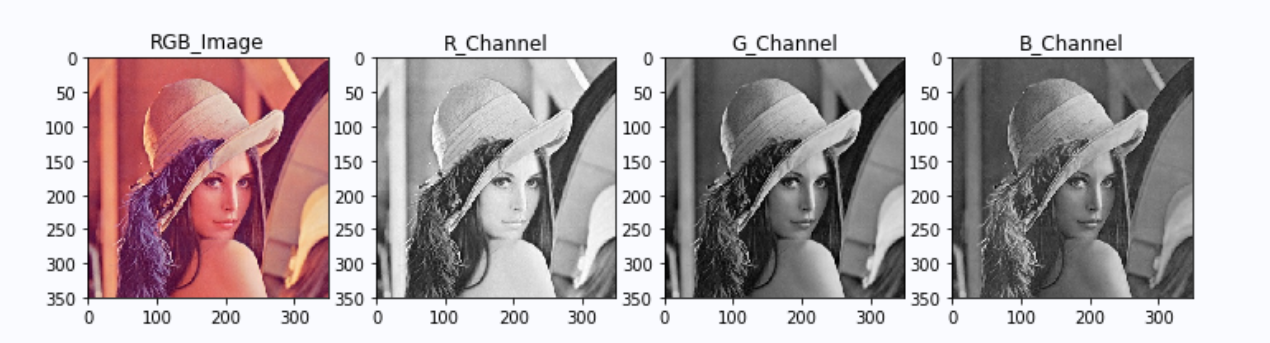# 二、图像的通道转换

## (一)、颜色空间转换

RGB或BGR到灰度（COLOR_RGB2GRAY，COLOR_BGR2GRAY）
RGB或BGR到YcrCb（或YCC）（COLOR_RGB2YCrCb，COLOR_BGR2YCrCb）
RGB或BGR到HSV（COLOR_RGB2HSV，COLOR_BGR2HSV）
RGB或BGR到Luv（COLOR_RGB2Luv，COLOR_BGR2Luv）

1. BGR图像转换为灰度图像
``````img = cv2.imread('lena.jpg')
# 转换为灰度图
img_gray = cv2.cvtColor(img, cv2.COLOR_BGR2GRAY)
# 保存灰度图
cv2.imwrite('img_gray.jpg', img_gray)
``````
1. GBR图像转换为RGB图像
``````# 加载彩色图
# 将彩色图的BGR通道顺序转成RGB
img = cv2.cvtColor(img, cv2.COLOR_BGR2RGB)
# 显示图片
plt.imshow(img)
#打印图片形状
print(img.shape)
``````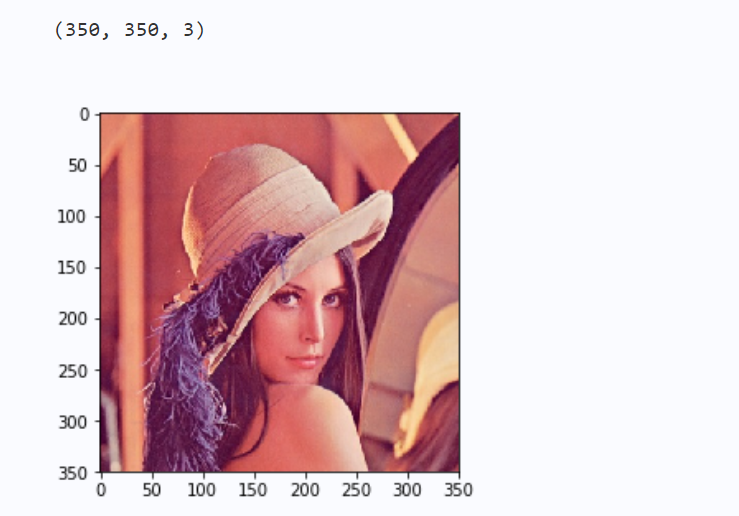## (二)、图片属性

1. 打印图片形状：
``````# 打印图片的形状
print(img.shape)
# 形状中包括行数、列数和通道数
height, width, channels = img.shape
print('图片高度：{}，宽度：{}，通道数：{}'.format(height,width,channels))
``````1. BGR转RGB
``````# 加载灰度图
# plt.imshow(img)

# 将彩色图的BGR通道顺序转成RGB
img = cv2.cvtColor(img, cv2.COLOR_BGR2RGB)
plt.imshow(img)

``````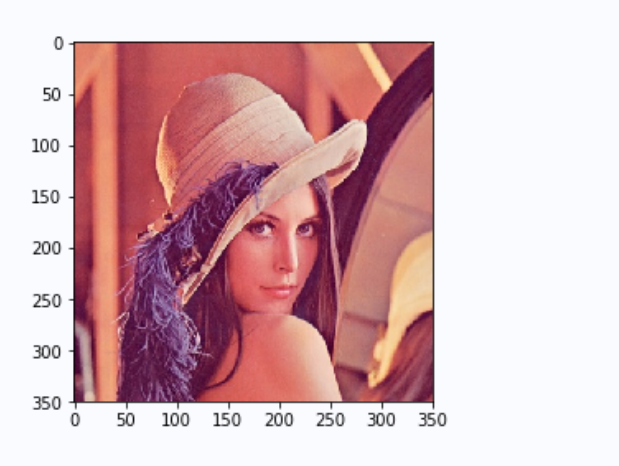1. 加载彩色图
``````# 加载彩色图
# 将彩色图的BGR通道直接转为灰度图
img = cv2.cvtColor(img, cv2.COLOR_BGR2GRAY)
plt.imshow(img,'gray')
img.shape
``````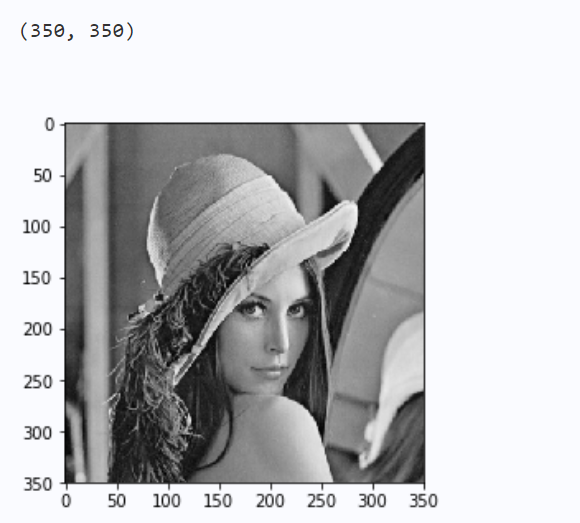# 三、图像拼接与缩放

## (一)、图像分割

``````%matplotlib inline
import numpy as np
import cv2
import matplotlib.pyplot as plt

path3 = 'test.jpg'

# the image height
sum_rows = img3.shape
print(img3.shape)
# print(sum_rows)
# the image length
sum_cols = img3.shape
# print(sum_cols)
part1 = img3[0:sum_rows, 0:int(sum_cols/2)]
print(part1.shape)
part2 = img3[0:sum_rows, int(sum_cols/2):sum_cols]
print(part2.shape)

plt.figure(figsize=(12,12))
#显示各通道信息
plt.subplot(121)
plt.imshow(part1)
plt.title('Image1')
plt.subplot(122)
plt.imshow(part2)
plt.title('Image2')

``````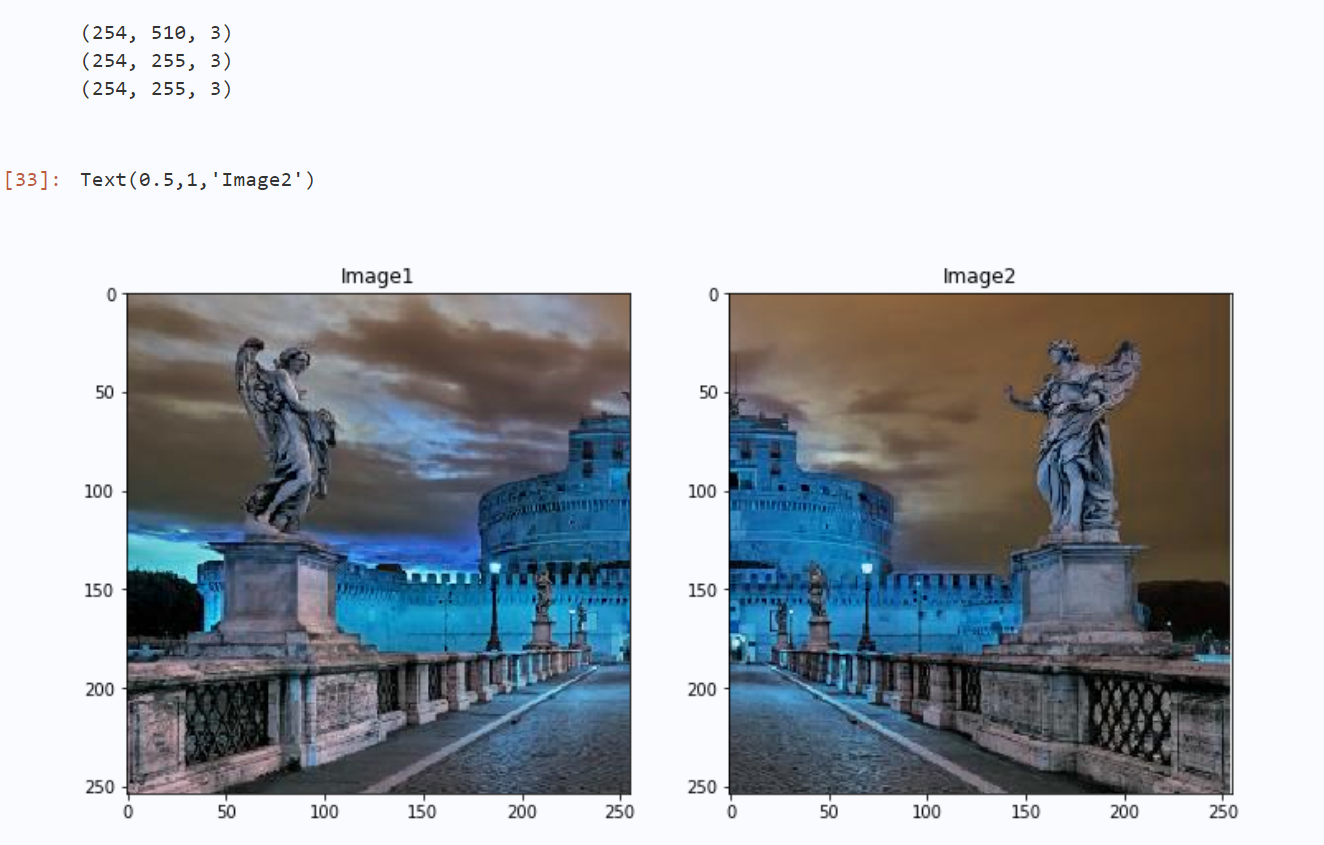## (二)、图像拼接

``````
img1 = part1
img2 = part2

# new image
final_matrix = np.zeros((254, 510, 3), np.uint8)
# change
final_matrix[0:254, 0:255] = img1
final_matrix[0:254, 255:510] = img2

plt.subplot(111)
plt.imshow(final_matrix)
plt.title('final_img')
``````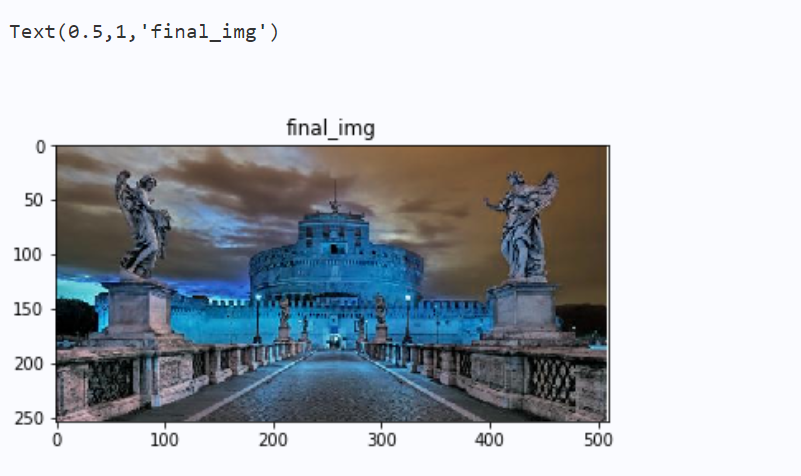## (三)、图像几何变换

OpenCV函数：cv2.resize(), cv2.flip(), cv2.warpAffine()

cv2.INTER_NEAREST 最近邻插值
cv2.INTER_LINEAR 线性插值
cv2.INTER_AREA 基于局部像素的重采样，区域插值
cv2.INTER_CUBIC 基于邻域4×4像素的三次插值
cv2.INTER_LANCZOS4 基于8×8像素邻域的Lanczos插值

1. 转换为RGB格式
``````img = cv2.imread('cat.png')
img = cv2.cvtColor(img, cv2.COLOR_BGR2RGB)
print(img.shape)
# 按照指定的宽度、高度缩放图片
res = cv2.resize(img, (400, 500))
plt.imshow(res)

``````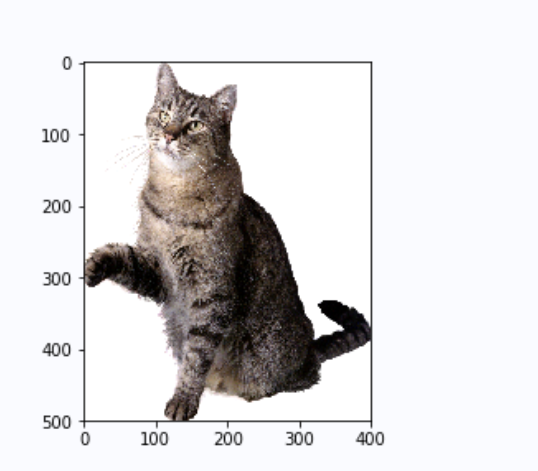1. 图像缩放
``````# 按照比例缩放，如x,y轴均放大一倍
res2 = cv2.resize(img, None, fx=5, fy=2, interpolation=cv2.INTER_LINEAR)
plt.imshow(res2)
``````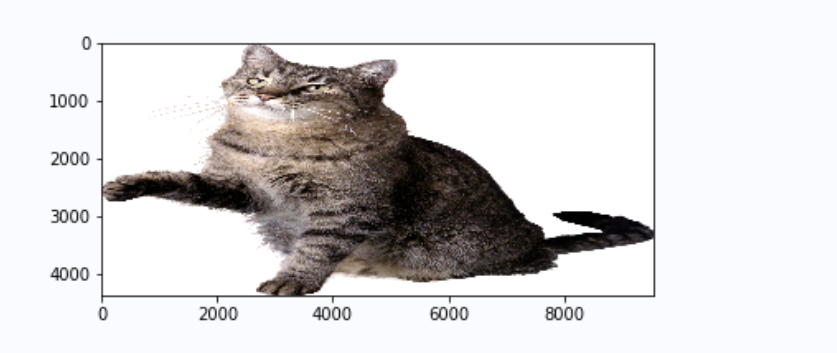# 四、图像二值化处理

• 使用固定阈值、自适应阈值和Otsu阈值法"二值化"图像
• OpenCV函数：`cv2.threshold()`, `cv2.adaptiveThreshold()`

### 阈值分割

`cv2.threshold()`用来实现阈值分割，ret是return value缩写，代表当前的阈值。函数有4个参数：

• 参数1：要处理的原图，一般是灰度图
• 参数2：设定的阈值
• 参数3：最大阈值，一般为255
• 参数4：阈值的方式，主要有5种，详情：ThresholdTypes
• 0: THRESH_BINARY 当前点值大于阈值时，取Maxval,也就是第四个参数，否则设置为0
• 1: THRESH_BINARY_INV 当前点值大于阈值时，设置为0，否则设置为Maxval
• 2: THRESH_TRUNC 当前点值大于阈值时，设置为阈值，否则不改变
• 3: THRESH_TOZERO 当前点值大于阈值时，不改变，否则设置为0
• 4:THRESH_TOZERO_INV 当前点值大于阈值时，设置为0，否则不改变
``````import cv2

# 灰度图读入
# 颜色通道转换
img = cv2.cvtColor(img, cv2.COLOR_BGR2RGB)
ret, th = cv2.threshold(img, 127, 255, cv2.THRESH_BINARY)
# 阈值分割

plt.imshow(th)
``````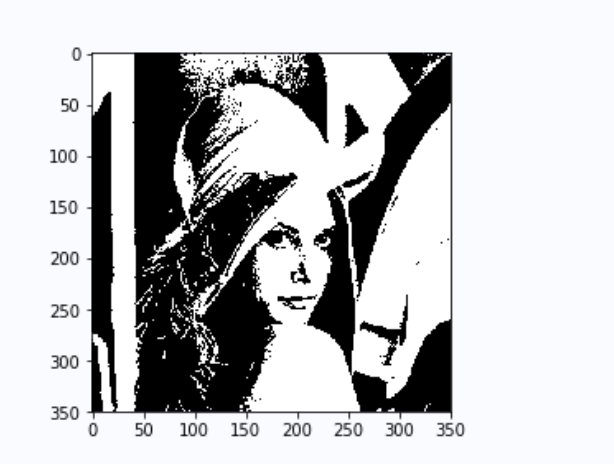# 五、图像归一化处理

• 知识点 01：均值（mean，average）

【解释】

1. 代表一组数据在分布上的集中趋势和总体上的平均水平；
2. 常说的中心化（Zero-Centered）或者零均值化（Mean-Subtraction），就是把每个数据都
减去均值；

【公式】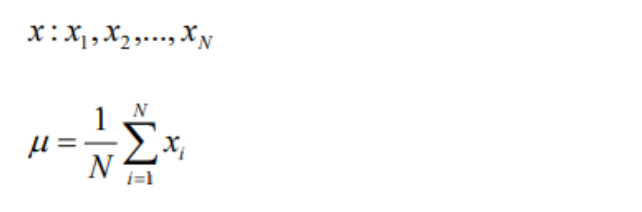• 知识点 02：标准差（Standard Deviation）

【解释】

1. 代表一组数据在分布上的离散程度；
2. 方差是标准差的平方

【公式】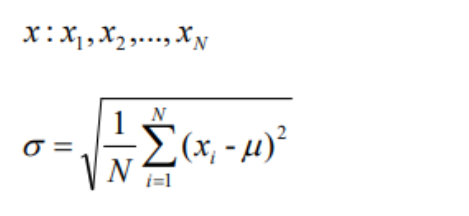``````import numpy as np
from PIL import Image
from paddle.vision.transforms import functional as F

img = np.asarray(Image.open('lena.jpg'))

mean = [0.31169346, 0.25506335, 0.12432463]
std = [0.34042713, 0.29819837, 0.1375536]
normalized_img = F.normalize(img, mean, std, data_format='HWC')

normalized_img = Image.fromarray(np.uint8(normalized_img))
plt.imshow(normalized_img)
``````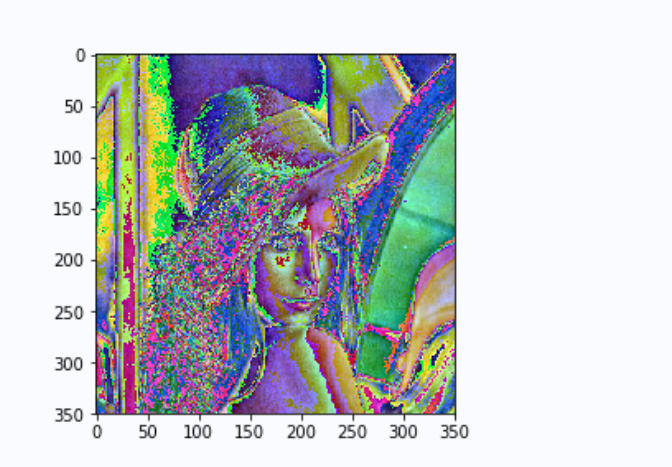# 总结

ps：更多精彩内容还请进入本文专栏人工智能，进行查看，欢迎大家支持与指教啊～(￣▽￣～)~(0)